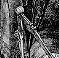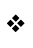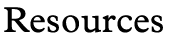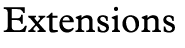Mathematical fns
Textual fns
Programming
Z-Machine
Glulx

Replacements

### Mathematical functions

Inform's mathematical abilities are ordinarily limited to signed 16-bit integer arithmetic, but these extensions change all that.

 fixedpt.h  (Version 1.0)  Matt Albrecht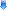Download To perform 16-bit fixed-point arithmetic longint.h  (Release 1)  Chris Hall and Francis IrvingDownload To perform signed and unsigned 32-bit integer arithmetic longint_dg.h    David GivenDownload To perform 16- and 32-bit integer arithmetic math.h  (Version 1.0)  Matt AlbrechtDownload Miscellaneous math functions mathpars.h  (Version 1.0)  Matt AlbrechtDownload For parsing big numbers (in decimal, hexadecimal or binary) random.h  (Version 1.3)  Roger FirthDownload A replacement for Inform's random function, which ensures well-distributed (and, where necessary, predictable) results, irrespective of which Z-machine or Glulx interpreter the player is using. A Z-Machine Floating Point Library  (1/1.020318)  Kevin Bracey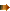Link To provide IEEE 754-conformant floating point arithmetic for the Z-machine (i.e., the standard platform on which Inform games run). Calculates to 9 decimal digits of accuracy with exponents from 10^99 to 10^(-99); translates floating point numbers to and from text. Fully supports rounding, subnormal numbers, infinity and exceptions.

Last updated 24 December 2007. This site is no longer supported; information may be out of date.
Maintained as a historical archive by the Interactive Fiction Technology Foundation. Copyright 1993-2018 IFTF, CC-BY-SA unless otherwise noted.